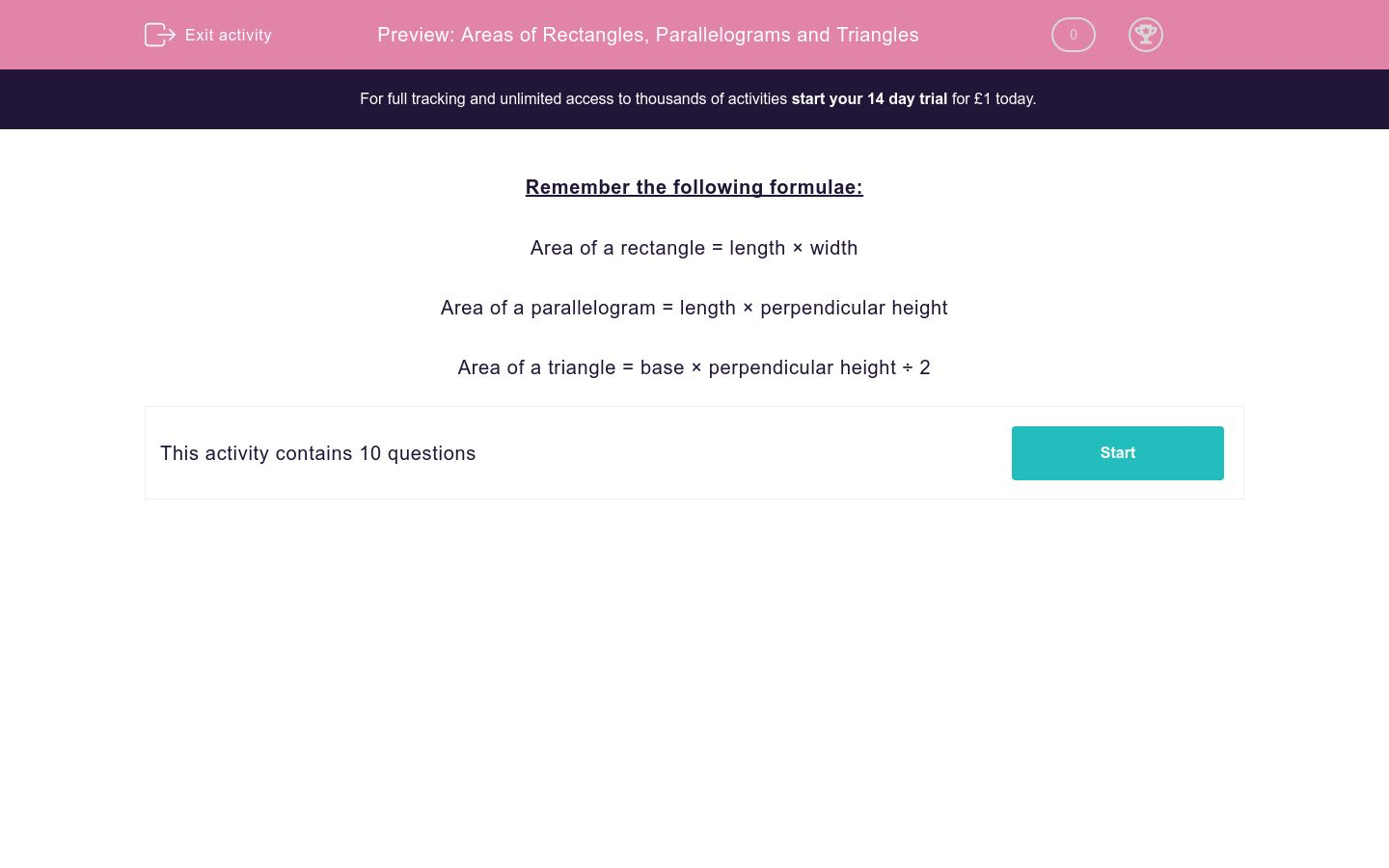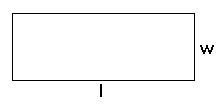# Areas of Rectangles, Parallelograms and Triangles

In this worksheet, students review how to find the areas of rectangles, parallelograms and triangles.Key stage:  KS 2

Curriculum topic:   Maths and Numerical Reasoning

Curriculum subtopic:   2D Shapes: Triangles, Quadrilaterals and Polygons

Difficulty level:### QUESTION 1 of 10

Remember the following formulae:

Area of a rectangle = length × width

Area of a parallelogram = length × perpendicular height

Area of a triangle = base × perpendicular height ÷ 2

Find the area of the following figure.h = 7 cm

b = 4 cm

Area =  __ cm2

Find the area of the following figure.h = 10 cm

l = 9 cm

Area =  __ cm2

Find the area of the following figure.l = 12 cm

w = 4.5 cm

Area =  __ cm2

Find the area of the following figure.h = 10 cm

l = 7 cm

Area =  __ cm2

Find the area of the following figure.h = 6 cm

l = 7 cm

Area =  __ cm2

Find the area of the following figure.w = 4 cm

l = 8.5 cm

Area =  __ cm2

Find the area of the following figure.h = 8 cm

l = 11 cm

Area =  __ cm2

Find the area of the following figure.h = 8 cm

l = 12 cm

Area =  __ cm2

Find the area of the following figure.h = 7 cm

b = 12 cm

Area =  __ cm2

Find the area of the following figure.h = 20 cm

b = 40 cm

Area =  __ cm2

• Question 1

Find the area of the following figure.h = 7 cm

b = 4 cm

Area =  __ cm2

14
• Question 2

Find the area of the following figure.h = 10 cm

l = 9 cm

Area =  __ cm2

90
• Question 3

Find the area of the following figure.l = 12 cm

w = 4.5 cm

Area =  __ cm2

54
• Question 4

Find the area of the following figure.h = 10 cm

l = 7 cm

Area =  __ cm2

70
• Question 5

Find the area of the following figure.h = 6 cm

l = 7 cm

Area =  __ cm2

42
• Question 6

Find the area of the following figure.w = 4 cm

l = 8.5 cm

Area =  __ cm2

34
• Question 7

Find the area of the following figure.h = 8 cm

l = 11 cm

Area =  __ cm2

88
• Question 8

Find the area of the following figure.h = 8 cm

l = 12 cm

Area =  __ cm2

96
• Question 9

Find the area of the following figure.h = 7 cm

b = 12 cm

Area =  __ cm2

42
• Question 10

Find the area of the following figure.h = 20 cm

b = 40 cm

Area =  __ cm2

400
---- OR ----

Sign up for a £1 trial so you can track and measure your child's progress on this activity.

### What is EdPlace?

We're your National Curriculum aligned online education content provider helping each child succeed in English, maths and science from year 1 to GCSE. With an EdPlace account you’ll be able to track and measure progress, helping each child achieve their best. We build confidence and attainment by personalising each child’s learning at a level that suits them.

Get started